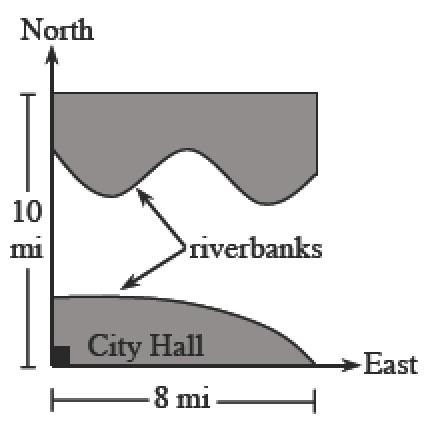### Home > APCALC > Chapter 7 > Lesson 7.2.2 > Problem7-67

7-67.

The city planning commission has set aside funds to build a bridge going north and south across the Newton River. To save money, they have agreed to build the shortest bridge possible. A map for the $8$-mile wide city is shown at right. The equations below represent the riverbanks where x is the number of miles east of City Hall and y is the number of miles north of City Hall. Determine the location for the shortest bridge that will span the Newton River.

North Bank: $y = \cos( \frac { \pi x } { 2 } )+6$ South Bank: $y = \ln(9 - x)$

At any give value of $x$, the distance between the North and South Banks is:

$y=\left ( \text{cos}\left ( \frac{\pi (x)}{2}\right )+6 \right )-(\text{ln}(9-x))$

Optimize.